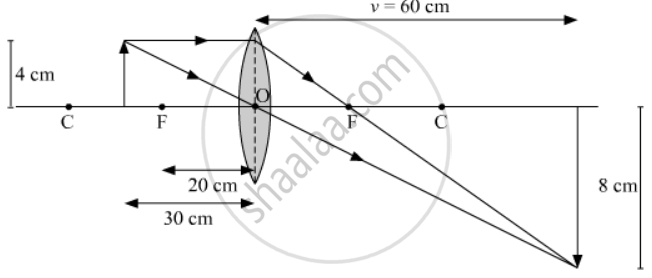# An Object of Height 4.0 Cm is Placed at a Distance of 30 Cm from the Optical Centre 'O' of a Convex Lens of Focal Length 20 Cm. Draw a Ray Diagram to Find the Position and Size of the Image Formed. - Science

An object of height 4.0 cm is placed at a distance of 30 cm from the optical centre 'O' of a convex lens of focal length 20 cm. Draw a ray diagram to find the position and size of the image formed. Mark optical centre 'O' and principal focus 'F' on the diagram. Also find the approximate ratio of size of the image to the size of the object.

#### Solution

Given that ,

u = -30 cm

f = 20 cm

v = ?

Using lens formula, we get

1/f = 1/v - 1/u

=> 1/20 = 1/v - 1/(-30)

=> 1/v = (3-2)/60

=> v = 60 cm

So the image will be formed at a distance of 60 cm from the lens as shown in the figure given below.Now using magnification formula we can write,

m = v/u = h_"image"/h_"object"

=> 60/(-30) = h_"image"/4

=> h_"image" = -8 cm

The negative sign shows that the image formed will be inverted and real. The ratio of size of the image to the size of the object is 8/4 = 2

Concept: Convex Lens
Is there an error in this question or solution?